# Analysis of the Performances of a Dish-stirling System Equipped with Hot Chamber

Analysis of the Performances of a Dish-stirling System Equipped with Hot Chamber

M. CucumoV. Ferraro D. Kaliakatsos M. Mele

Department of Mechanical, Energetics and Management Engineering (DIMEG), University of Calabria – Via P. Bucci - 87036 Rende (CS) – Italy

Corresponding Author Email:
m.cucumo@unical.it
Page:
125-136
|
DOI:
http://dx.doi.org/10.18280/ijht.330416
|
Accepted:
|
Published:
31 December 2015
| Citation

OPEN ACCESS

Abstract:

This paper analyses the performances of a Dish-Stirling concentrating solar system using a Stirling engine for the production of electrical and thermal energy. In particular, with focus on the receiver, its performance was evaluated considering two different plant solutions: “naked” receiver (without hot chamber) and receiver provided with hot chamber.

The Dish-Stirling system under study includes a MICROGEN linear piston Stirling engine of 4 kW total rated power (3 kW thermal and 1 kW electric). The minimum engine starting temperature is 190 °C, while the maximum operating temperature is 565 °C. The analysis is developed through the setting up of a calculation model in MatLab, through which it is possible to predict the dynamic behaviour of the concentrator-receiver-Stirling engine system and quantify the energy productivity in various operating conditions. The simulations results show that the use of a hot chamber allows improvement of the system performance which, as regards the thermal power, is quantified in an increase of over 70%.

(Presented at the AIGE Conference 2015)

Keywords:

Dish-Stirling, Hot chamber, Thermal-electric performance.

1. Introduction

Electrical and thermal energy can be produced, for small users, by means of solar thermal technology at medium-high temperature (CSP technologies, Concentrating Solar Power) such as the “parabolic dish focus point” technology. Direct solar radiation is collected on a reflective surface formed by parabolic mirrors and focused on the focal point; the mirrors track the sun with a rotation movement about two orthogonal axes, in order to collect all the direct components of the radiation throughout the day; the heat at a high temperature is transferred to a fluid and used in a Stirling engine, placed in contact with the absorber, to produce electrical and thermal energy directly. The Dish-Stirling system has already been produced and marketed in Italy .

In this work a mathematical model has been developed to simulate the absorber of the Dish-Stirling system equipped with hot chamber, in order to analyse the system performance in relation to its electrical and thermal energy production. The hot chamber has the function of creating a sort of black cavity: the solar rays, impacting on the dish, are concentrated towards the entrance of the hot chamber hole in which, apart from small losses, they remain confined and absorbed after a series of reflections and absorptions. In this way, therefore, only a very small part of the concentrated energy is able to escape from the chamber-absorber system: this energy is that of the rays which, once reflected, again escape through the hole.

In this work in order to test the improvement in performances resulting from  the  use  of  the  hot  chamber, a

comparison between a system with  absorber  without  hot chamber and a system with absorber provided with hot chamber was performed.

2. the Dish-stirling System

Figure 1 shows the layout of the Dish-Stirling system analysed.

## 1.pngFigure 1. Layout of the Dish-Stirling system

The concentrator of a CSP system is made from reflective materials able to direct most of the incident solar rays onto it with minimum optical losses. The reflective surface is generally made of aluminium or of silver deposited on glass or plastic plates.

The reflection ρp and convergence F coefficients of the parabola were assumed, respectively, at 0.89 and 0.98. The geometric form that ensures minimal optical losses for this type of application is the revolution paraboloid, which, in this case, has a collecting area of 10 m2.

The receiver, the element of the concentration system that absorbs the solar rays and transfers the heat to the working fluid of the Stirling engine, has a trunk-conical geometry as shown in Figure 2.

The head of the Stirling engine is housed in the cavity of the receiver. The heat transfer between the receiver and the head of the engine is by conduction, once the solar rays have been absorbed (Figure 3).

In this study, the engine used in the concentration system is a linear piston Stirling engine with a total rated output of 4 kW. Figure 4 shows the characteristic curves of the engine supplied by the manufacturer.

## 2.png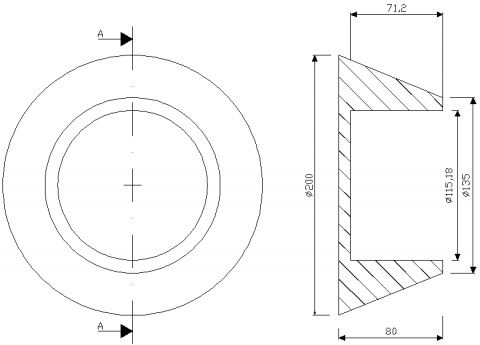Figure 2. Representation of the receiver

## 3.png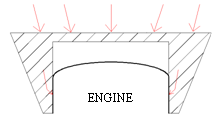## 4.png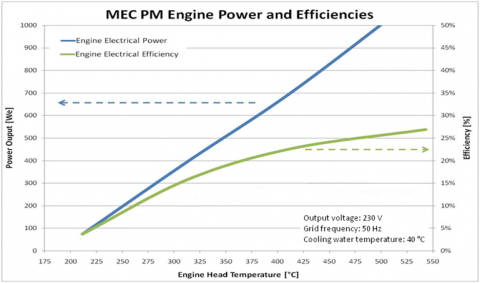Figure 4. Stirling Engine characteristic curves

From the same figure, it is also possible to deduce the range of temperature in which the engine operates: the minimum ignition temperature is 190 °C, and the maximum temperature, beyond which the engine may suffer irreversible deformations, is 565 °C.

From the experimental curves provided by the manufacturer, polynomial Eq.s were deduced according to the absorber temperature Tass, to calculate the electrical power produced by the engine and the total thermal power absorbed:

$P_{\mathrm{el}}=2.865 \cdot \mathrm{T}_{\mathrm{ass}}-532.5$      (1)

$\mathrm{Q}_{\mathrm{u}}=\mathrm{a} \cdot \mathrm{T}_{\mathrm{ass}}^{6}+\mathrm{b} \cdot \mathrm{T}_{\mathrm{ass}}^{5}+\mathrm{c} \cdot \mathrm{T}_{\mathrm{ass}}^{4}+\mathrm{d} \cdot \mathrm{T}_{\mathrm{ass}}^{3}+\mathrm{e} \cdot \mathrm{T}_{\mathrm{ass}}^{2}+\mathrm{f} \cdot \mathrm{T}_{\mathrm{ass}}+\mathrm{g}$     (2)

The coefficients of Eq.(2) are shown in Table 1.

Table 1. Coefficients of Eq.(2)

 a 4.10068e-12 b -1.08525e-08 c 1.18255e-05 d -0.00679968 e 2.1932 f -373.113 g 28026.3

Unlike the traditional Dish-Stirling system, the system under study is characterized by an absorber enclosed in a hot chamber in order to achieve the characteristics of a black cavity and increase the performance of the entire plant    (Figure 5).

## 5.pngFigure 5. Receiver provided with hot chamber

3. Calculation Models

In the work the Eq.s are presented that describe the physical phenomenon and the calculation model, developed in a MatLab environment, through which the dynamic behaviour of the concentrator-receiver-Stirling engine system can be predicted to quantify the energy productivity. Two system solutions are compared: the first that uses the absorber with hot chamber and the second with simple absorber.

3.1 Absorber equipped with hot chamber

In order to assess the temperature reached by the absorber and the hot chamber, a model discretization in three walls was used (Figure 6): the outer surface of the absorber (wall 1), the inner surface of the hot chamber (wall 2) and the surface of the inlet hole of the hot chamber (wall 3).

## 6.png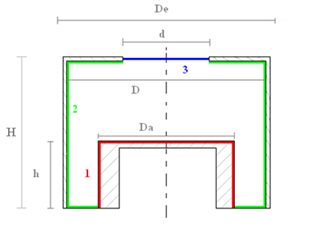Figure 6. Discretization in three walls of the absorber and hot chamber

Compared to the real model, a simplification was made to the shape of the absorber which was considered cylindrical rather than trunk-conical in shape.

Referring to Figure 6, the following parameters were defined: De outer diameter of the chamber, d diameter of the inlet hole, D inner diameter of the chamber, Da absorber diameter, H chamber height and h absorber height.

The analysis was carried out by running the energy balances on the different walls in the solar spectrum to short wavelength, and in the infrared spectrum at high wavelength.

Analysis in the solar spectrum. The radiance of the surfaces forming the cavity, in the solar spectrum, are made solely by the terms of the radiation reflected from the surfaces because they can not emit in this spectrum:

$\mathrm{J}_{\mathrm{j}}^{\mathrm{c}}=\rho_{\mathrm{j}}^{\mathrm{c}} \sum_{\mathrm{k}=1}^{\mathrm{n}} \mathrm{F}_{\mathrm{jk}} \mathrm{J}_{\mathrm{k}}^{\mathrm{c}}$     (3)

In Eq.(3), ρjc is the reflection coefficient of the “j” surface and Fjk is the view factor between the surfaces “j” and “k”.

Solar radiation entering the chamber through the hole, for an observer within it, can be interpreted as the radiance of the same hole. It is known a priori and is equal to the incident flux on the receiver:

$\mathrm{J}_{\mathrm{f}}^{\mathrm{c}}=\frac{\mathrm{DNI} \cdot\left(\mathrm{A}_{\mathrm{p}}-\mathrm{A}_{\mathrm{o}}\right) \cdot \cos (\mathrm{i}) \cdot \rho_{\mathrm{p}} \cdot \mathrm{F}}{\mathrm{A}_{\mathrm{h}}}$   (4)

In Eq.(4), DNI is the direct normal radiation to the ground, Ap and Ao represent, respectively, the total area of the concentrator and the area fraction which does not receive radiation due to the shadow projected on it from the hot chamber, i is the angle of incidence of solar radiation (to take into account a hypothetical error of the tracking system, an angle of incidence of solar radiation of 0.3° was hypothesized), ρp is the reflection coefficient of the parabola, F is the convergence coefficient and Ah is the area of the hole of the hot chamber.

The view factors are determined by the geometry of the system and are valued using the principle of reciprocity and the additive property .

The direct normal radiation to the ground was calculated by Eq.(5) according to the ASHRAE model  to estimate the radiation to the ground on a clear day:

$\mathrm{DNI}=A \cdot \exp \left(-\frac{\mathrm{B}}{\sin \beta}\right)$      (5)

Where β is the angle of solar height, A the apparent solar radiation outside of the atmosphere and B is the atmospheric extinction coefficient. Their values, for the twenty-first day of each month, are shown in Table 2.

Table 2. Values of the constants A and B for the ASHRAE model

 Month A [W/m2] B [-] Jan. 1 230 0.142 Feb. 1 215 0.144 Mar. 1 186 0.156 Apr. 1 136 0.180 May 1 104 0.196 June 1 088 0.205 July 1 085 0.207 Aug. 1 107 0.201 Sept. 1 151 0.177 Oct. 1 192 0.160 Nov. 1 221 0.149 Dec. 1 233 0.142

After determining the radiance by the above Eq.s, the solar flux absorbed by each wall can be evaluated:

$\Phi_{\mathrm{ass}, j}^{\mathrm{c}}=\alpha_{\mathrm{j}}^{\mathrm{c}} \cdot \sum_{\mathrm{k}=1}^{\mathrm{n}} \mathrm{F}_{\mathrm{jk}} \cdot \mathrm{J}_{\mathrm{k}}^{\mathrm{c}}$     (6)

Where αjc is the absorption coefficient of j-th surface in the solar spectrum, which is the complement to one of the corresponding reflection coefficients.

$\alpha_{j}^{c}=1-\rho_{j}^{c}$      (7)

The flow $\Phi_{\mathrm{ass}, \mathrm{f}}$ is, in reality, a “fictitious absorbed flow”; in fact, it represents the fraction of energy that is again sent outside through the hole (the absorption coefficient of the hole is unitary).

Analysis in the infrared spectrum. The walls, after absorbing solar radiation and their temperature is increased, re-emit energy in the infrared spectrum. In this spectrum, the radiance contains both the emitted and the reflected component. For the j-th surface radiance is written:

$\mathrm{J}_{\mathrm{j}}^{1}=\varepsilon_{\mathrm{j}}^{1} \sigma \mathrm{T}_{\mathrm{j}}^{4}+\left(1-\varepsilon_{\mathrm{j}}^{1}\right) \cdot \sum_{\mathrm{k}=1}^{\mathrm{n}} \mathrm{F}_{\mathrm{jk}} \mathrm{J}_{\mathrm{k}}^{1}$      (8)

Where $T_{a}$ is the infrared emissivity of j-th surface and σ is the Stefan-Boltzmann constant.

The incoming radiance through the hole, since it is not provided with glass, is assumed equal to:

$\mathrm{J}_{f}^{1}=\sigma \cdot \mathrm{T}_{\mathrm{a}}^{4}$     (9)

Where Ta  is the outside air temperature.

The flows absorbed in high wavelength are dependent on contributions from the energy incident on the surface under examination from the other surfaces of the cavity. Assuming that all the surfaces are grey, the following relationship holds:

$\Phi_{\mathrm{ass}, \mathrm{j}}^{1}=\alpha_{\mathrm{j}}^{1} \sum_{\mathrm{k}=1}^{\mathrm{n}} \mathrm{F}_{\mathrm{jk}} \mathrm{J}_{\mathrm{k}}^{1}=\varepsilon_{\mathrm{j}}^{1} \sum_{\mathrm{k}=1}^{\mathrm{n}} \mathrm{F}_{\mathrm{jk}} \mathrm{J}_{\mathrm{k}}^{1}$     (10)

Overall balance of the surface. In order to determine the temperatures reached by the absorber Tass (wall 1) and the hot chamber Tc (wall 2), it is necessary to analyse the overall energy balance of the walls, which takes contributions in the two spectra into account.

Therefore the energy balance is the following:

$\left\{\begin{array}{l}\frac{\mathrm{Q}_{\mathrm{u}}}{\mathrm{A}_{1}}+\varepsilon_{1}^{1} \cdot \sigma \cdot \mathrm{T}_{\mathrm{ass}}^{4}-\Phi_{\mathrm{ass}, 1}^{\mathrm{c}}-\Phi_{\mathrm{ass}, 1}^{1}=0 \\ \frac{\mathrm{Q}_{\mathrm{p}}}{\mathrm{A}_{2}}+\varepsilon_{2}^{1} \cdot \sigma \cdot \mathrm{T}_{\mathrm{c}}^{4}-\Phi_{\mathrm{ass}, 2}^{\mathrm{c}}-\Phi_{\mathrm{ass}, 2}^{1}=0 \\ \mathrm{J}_{1}^{1}=\varepsilon_{1}^{1} \sigma \mathrm{T}_{\mathrm{ass}}^{4}+\left(1-\varepsilon_{1}^{1}\right)\left[\mathrm{F}_{12} \mathrm{J}_{2}^{1}+\mathrm{F}_{13} \mathrm{J}_{3}^{1}\right] \\ \mathrm{J}_{2}^{1}=\varepsilon_{2}^{1} \sigma \mathrm{T}_{\mathrm{c}}^{4}+\left(1-\varepsilon_{2}^{1}\right)\left[\mathrm{F}_{21} \mathrm{J}_{1}^{1}+\mathrm{F}_{22} \mathrm{J}_{2}^{1}+\mathrm{F}_{23} \mathrm{J}_{3}^{1}\right]\end{array}\right.$      (11)

The power Qp, given by the hot chamber, is the sum of two contributions: power lost to the outside by convection and radiation (negligible because of the outer insulation of the chamber) and cooling power of the hot chamber walls Qw, different from zero if the temperature reached by the absorber is greater than the maximum value allowed for the proper functioning of the Stirling engine.

The code set up allows performance of the analysis in the solar spectrum, necessary for the assessment of the flows absorbed at low wavelength from the two surfaces constituting the cavity ( $\Phi_{\mathrm{ass}, 1}$ , $\Phi_{\mathrm{ass}, 2}^{\mathrm{c}}$ ), and subsequently the analysis in the infrared spectrum, to assess the temperatures reached by absorber and hot chamber.

In order to ensure integrity and functionality of the engine, the hot chamber is provided with a cooling system. The function of this system is to intervene when the temperature reached by the absorber exceeds the maximum allowed. Drawing appropriate power Qw through cooling of the chamber surfaces, the temperature of the absorber is always kept at the maximum value and the system can provide the nominal values of electrical power. In this case, the Eq.s to be solved are the same as those described by the Eq. system (11), in which, however, the temperature Tass (set equal to 565 °C) is no longer unknown but the power Qw included in Qp.

In addition, the control system used provides the partial defocusing of the parabola which should not be able to maintain the temperature of the absorber lower than 565 °C, despite the cooling of the chamber surfaces.

3.2 Absorber without hot chamber

For the calculation of the temperature reached by the absorber in the absence of a hot chamber, it must satisfy the Eq. of energy balance, Eq.(12), in which the power absorbed by the receiver must equal the useful power supplied by the same, that is, the power absorbed by the Stirling engine, Eq.(2), and the power from it lost by convection and radiation.

$\mathrm{P}_{\mathrm{a}_{-} \mathrm{ric}}=\mathrm{P}_{\mathrm{p}_{-} \mathrm{con}}+\mathrm{P}_{\mathrm{p}_{-}} \operatorname{irr}+\mathrm{Q}_{\mathrm{u}}$      (12)

The power absorbed by the receiver, which represents a rate of solar energy incident on the parabola, and the losses due to convection and radiation of the same, can be expressed through the relationship:

$\mathrm{P}_{\mathrm{a}_{-} \mathrm{ic}}=\mathrm{DNI} \cdot\left(\mathrm{A}_{\mathrm{p}}-\mathrm{A}_{0}\right) \cdot \cos (\mathrm{i}) \cdot \rho_{\mathrm{p}} \cdot \mathrm{F} \cdot \alpha_{1}^{\mathrm{c}}$      (13)

$P_{p_{-} c o n}=\left(A_{f}+A_{l}\right) \cdot h_{c} \cdot\left(T_{a s s}-T_{a}\right)$     (14)

$P_{p_{-} \text {irr }}=\left(A_{f}+A_{1}\right) \cdot \varepsilon_{1}^{1} \cdot \sigma \cdot\left(T_{\text {ass }}^{4}-T_{a}^{4}\right)$   (15)

In which Af and Al indicate, respectively, the front and the side area of the absorber, while hc is the convective heat transfer coefficient, assumed at 20 Wm-2K-1. In accordance with the numbering shown in Figure 6, the subscript 1 was used for the emissivity and for the absorptivity of the absorber (in this case there is no wall 2).

3.3 The storage tank

In order to meet better the user requirements, the solar system is usually accompanied by a storage tank. The temperature of the tank is calculated by solving the following energy balance:

$P_{\text {ter }}-Q_{los s}=\frac{\rho_{a} V_{s} c_{p}}{\Delta t}\left(T_{s}^{t}-T_{s}^{t-\Delta t}\right)$     (16)

In Eq.(16), ρa is the density of water, Vs the volume of the tank, \Delta t  the number of seconds between a time instant and the previous one, while Ts is the tank temperature.

The power lost from the tank, Qloss, is calculated by the following Eq.:

$\mathrm{Q}_{ \mathrm{loss}}=\mathrm{H}_{\mathrm{s}} \cdot \mathrm{S}_{\mathrm{s}} \cdot\left(\mathrm{T}_{\mathrm{s}}^{\mathrm{t}}-\mathrm{T}_{\mathrm{a}}\right)$    (17)

In Eq.(17), Hs and Ss are, respectively, the transmittance and the heat exchange surface of the tank. In the simulations a 1000-litre storage tank was used. The water temperature in the storage tank, at starting up of the system, was set at 20 °C.

The total thermal power Pter is sum of the power cooling of the chamber surfaces Qw (in the ranges where it is cooled), and the cooling power of the engine Pt_m.

The latter is calculated by Eq.(18).

$\mathrm{P}_{\mathrm{t}_{-} \mathrm{m}}=\mathrm{Q}_{\mathrm{u}}-\mathrm{P}_{\mathrm{loss}}-\mathrm{P}_{\mathrm{el}}$   (18)

In Eq.(18) the power loss from the engine Ploss is a data provided by the manufacturer and it is equal to 300 W.

3.4 Factors characterizing the system performances

Once determined the temperature of the absorber, the electrical power produced by the engine and the total thermal power consumption by applying Eqs. (1) and (2) can be calculated.

The incident power on the parabola Pi_par, the incident power on the receiver Pi_ric, and the related efficiencies ηpar and ηric, are evaluated with following Eq.s:

$P_{i_{-} \text {par }}=D N I \cdot\left(A_{p}-A_{0}\right) \cdot \cos (i)$  (19)

$P_{1_{-} \operatorname{icc}}=\operatorname{DNI} \cdot\left(A_{p}-A_{0}\right) \cdot \cos (i) \cdot \rho_{p} \cdot F$   (20)

$\eta_{\mathrm{par}}=\frac{P_{i_{-} \mathrm{ric}}}{P_{i_{-} p a r}}$    (21)

$\eta_{\mathrm{ric}}=\frac{Q_{\mathrm{u}}}{P_{\mathrm{i}_{-} \mathrm{ric}}}$    (22)

To define the system performance in both configurations (absorber with or without hot chamber), reference is made to dimensionless parameters that characterize the electrical and thermal production. These parameters are the electrical ηel, thermal ηter and total ηg efficiency (according with first and second thermodynamics laws), evaluated at the system or engine level.

For the system:

$\eta_{\mathrm{el}}=\frac{\mathrm{P}_{\mathrm{el}}}{\mathrm{P}_{\mathrm{i}_{\mathrm{par}}}}$     (23)

$\eta_{\mathrm{ter}}=\frac{P_{\mathrm{ter}}}{P_{\mathrm{i}_{-} \mathrm{par}}}$   (24)

$\eta_{\mathrm{g}, \mathrm{IP}}=\frac{P_{\mathrm{ter}}+P_{\mathrm{el}}}{P_{\mathrm{i}_{-} \mathrm{par}}}$    (25)

$\eta_{\mathrm{g}, \mathrm{IIP}}=\frac{P_{\mathrm{ter}} \cdot \tau_{1}+P_{\mathrm{el}}}{\mathrm{P}_{\mathrm{i-par}}}$    (26)

For the engine:

$\eta_{\mathrm{el}, \mathrm{m}}=\frac{\mathrm{P}_{\mathrm{el}}}{\mathrm{Q}_{\mathrm{u}}}$  (27)

$\eta_{\mathrm{ter}, \mathrm{m}}=\frac{\mathrm{P}_{\mathrm{t}_{-} \mathrm{m}}}{\mathrm{Q}_{\mathrm{u}}}$      (28)

$\eta_{g, m, I P}=\frac{P_{t_{-} m}+P_{e l}}{Q_{u}}$   (29)

$\eta_{\mathrm{g}, \mathrm{m}, \mathrm{IIP}}=\frac{\mathrm{P}_{\mathrm{t} \mathrm{m}} \cdot \tau_{1}+\mathrm{P}_{\mathrm{el}}}{\mathrm{Q}_{\mathrm{u}}}$    (30)

The Carnot factor τ1 or dimensionless exergetic temperature , is calculated between the maximum allowable temperature of the engine and the ambient temperature.

4. Comparison Between the Two System Solutions

In Figures 7 and 8 the graphical interfaces of the calculation models, implemented in MatLab, respectively for the system with a hot chamber and for the system with simple absorber are reported.

Cosenza was chosen as reference location (L = 39.3°, l = 16.15 °E). The simulations were performed for a full year of system operation.

The characteristic geometry data of the system are reported in Table 3. The lateral surface of the absorber (in the absence of chamber) and the hot chamber are insulated with 5 cm of insulating material with a thermal conductivity of               0.04 Wm-2K-1. They were considered two different types of absorber in relation to the material used: absorber in sandblasted stainless steel (Ass_Inox) and absorber in Silicon Carbide (Ass_SiC). In both cases the Alanod was used for the hot chamber as characterized by high values of reflectivity.

Table 4 reports the absorption coefficient, in the solar spectrum, and the emittance, in the infrared spectrum, of the materials used in the simulations.

## 7.png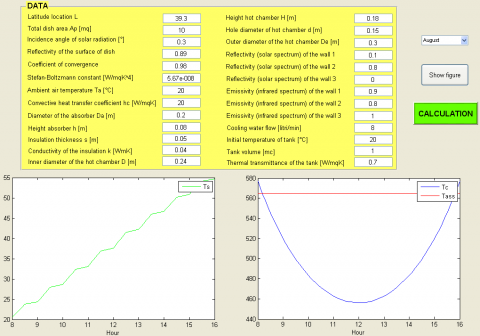Figure 7. Graphical interface of the computer code for the system with hot chamber

## 8.png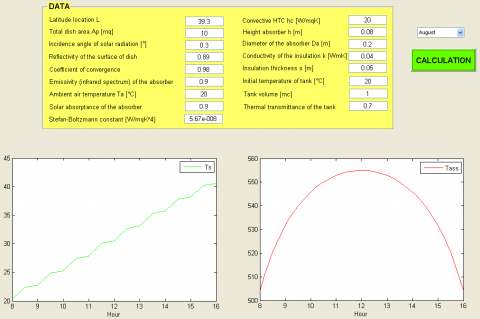Figure 8. Graphical interface of the computer code for the system without hot chamber

Table 3. Geometric data for absorber and hot chamber

 Da [m] 0.2 h [m] 0.08 H [m] 0.18 D [m] 0.24 d [m] 0.15 De [m] 0.3

Table 4. Optical characteristics of the materials used

 αc Absorber 0.78 - Stainless steel oxidized (sandblasted) 0.90 - Silicon carbide (SiC) Hot chamber 0.20 - Alanod Hole 1.00 εl Absorber 0.60 - Stainless steel oxidized  (sandblasted) 0.90 - Silicon carbide (SiC) Hot chamber 0.80 - Alanod Hole 1.00

The simulation results obtained for the absorber in stainless steel, are shown in Tables 5 and 6. In the latter, in particular, the various efficiencies assessed at the level of the overall system are reported.

Table 7 shows the average annual efficiency of the engine in the case of stainless steel absorber. The efficiency of receiver is equal to 62% if it is provided with hot chamber and to 55.3% in the contrary case.

In Figures 9-17, for the absorber in SiC, with and without hot chamber, the trend of the absorber temperature Tass, of the absorbed power of the same Pa_ric, of the power absorbed by the engine Qu, of the electric power of the motor Pel, of thermal power Pter, of thermal efficiency ηter, of electrical efficiency ηel, of the total efficiency of the first ηg,IP and second law ηg,IIP, of the efficiency of the absorber ηric, evaluated for the system, are presented.

Table 5. Values of Tass, Pa_ric, Qu, Pel, Pter (Ass_Inox)

 Month Model Tass [°C] Pa_ric   [W] Qu    [W] Pel    [W] Pter   [W] Jan. Chamber 551.23 6 386.13 4 419.89 1 046.78 4 533.32 No chamber 496.70 5 418.33 3 860.43 890.54 2 669.89 Feb. Chamber 564.63 7 009.17 4 561.72 1 085.15 5 189.66 No chamber 531.78 5 946.95 4 196.33 991.05 2 905.28 Mar. Chamber 565.00 7 226.39 4 566.12 1 086.23 5 453.76 No chamber 542.88 6 131.25 4 313.94 1 022.86 2 991.08 Apr. Chamber 565.00 7 020.34 4 566.12 1 086.23 5 197.20 No chamber 533.34 5 956.42 4 204.12 995.52 2 908.60 May Chamber 565.00 6 856.31 4 566.12 1 086.23 4 994.34 No chamber 525.51 5 817.25 4 116.13 973.07 2 843.06 June Chamber 565.00 6 741.73 4 566.12 1 086.23 4 853.04 No chamber 519.92 5 720.03 4 054.40 957.06 2 797.34 July Chamber 565.00 6 681.24 4 566.12 1 086.23 4 778.58 No chamber 516.91 5 668.71 4 021.70 948.44 2 773.26 Aug. Chamber 565.00 6 734.57 4 566.12 1 086.23 4 844.41 No chamber 519.46 5 713.96 4 050.35 955.77 2 794.59 Sept. Chamber 565.00 6 940.25 4 566.12 1 086.23 5 098.62 No chamber 529.33 5 888.47 4 160.79 984.04 2 876.75 Oct. Chamber 564.82 6 860.11 4 564.05 1 085.72 5 002.54 No chamber 524.81 5 820.47 4 116.69 971.09 2 845.60 Nov. Chamber 555.38 6 444.10 4 460.82 1 058.65 4 571.60 No chamber 501.27 5 467.51 3 891.30 903.63 2 687.67 Dec. Chamber 540.11 6 115.28 4 322.72 1 014.92 4 281.25 No chamber 479.29 5 188.52 3 730.52 840.66 2 589.86 Mean values Chamber 560.93 6 751.30 4 524.34 1 074.57 4 899.86 No chamber 518.43 5 728.16 4 059.73 952.81 2 806.92

Table 6. Values of ηter, ηel, ηg,IP, ηg,IIP   (Ass_Inox)

 Month Model ηter ηel ηg,IP ηg,IIP Jan. Chamber 0.572 0.132 0.705 0.504 No chamber 0.335 0.112 0.447 0.330 Feb. Chamber 0.597 0.125 0.722 0.513 No chamber 0.332 0.113 0.446 0.329 Mar. Chamber 0.609 0.121 0.730 0.517 No chamber 0.332 0.113 0.445 0.329 Apr. Chamber 0.597 0.125 0.722 0.513 No chamber 0.332 0.114 0.446 0.330 May Chamber 0.587 0.128 0.715 0.510 No chamber 0.332 0.114 0.446 0.330 June Chamber 0.580 0.130 0.710 0.507 No chamber 0.333 0.114 0.447 0.330 July Chamber 0.577 0.131 0.708 0.506 No chamber 0.333 0.114 0.447 0.330 Aug. Chamber 0.580 0.130 0.710 0.507 No chamber 0.333 0.114 0.447 0.330 Sept. Chamber 0.592 0.126 0.719 0.511 No chamber 0.332 0.114 0.446 0.330 Oct. Chamber 0.588 0.128 0.716 0.510 No chamber 0.333 0.114 0.446 0.330 Nov. Chamber 0.572 0.132 0.716 0.504 No chamber 0.334 0.112 0.447 0.330 Dec. Chamber 0.564 0.134 0.698 0.501 No chamber 0.340 0.110 0.450 0.331 Mean values Chamber 0.585 0.128 0.714 0.509 No chamber 0.333 0.113 0.447 0.330

Table 7. Mean values of  ηter,m, ηel,m, ηg,m,IP, ηg,m,IIP (Ass_Inox)

 ηter,m ηel,m ηg,m,IP ηg,m,IIP Mean values With chamber 0.696 0.237 0.934 0.690 Without chamber 0.691 0.235 0.926 0.684

Tables 8 and 9 show the average annual values of parameters obtained using the absorber in SiC.

Table 10 reports the values of the average annual efficiency of the engine in the case of SiC absorber.

Table 11 shows the percentage differences, for the two absorbers, among the average values of the variables obtained for the system with hot chamber and those obtained for the system without hot chamber.

## 9.png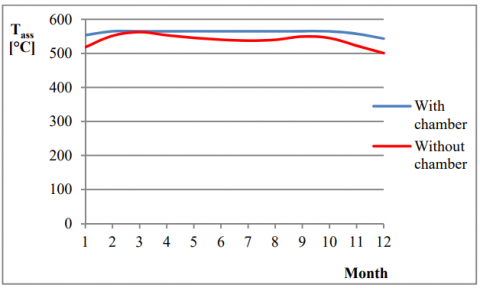Figure 9. Absorber temperature (Ass_SiC)

## 10.png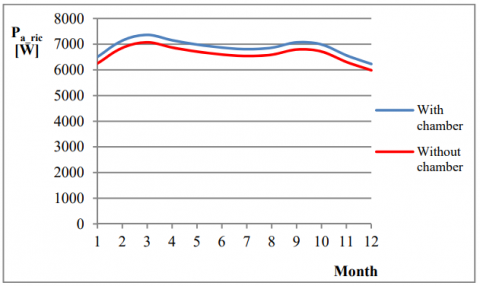Figure 10. Power absorbed from absorber (Ass_SiC)

## 11.pngFigure 11. Power absorbed from engine (Ass_SiC)

## 12.png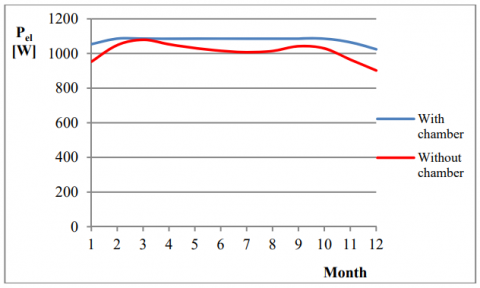Figure 12. Electrical power of engine (Ass_SiC)

## 13.png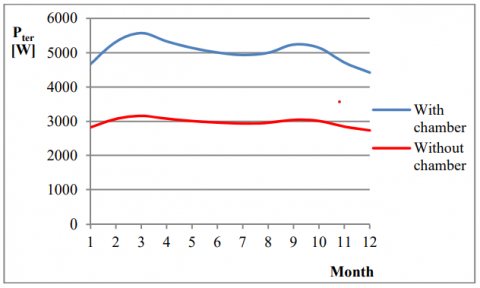Figure 13. Total thermal power of the system (Ass_SiC)

## 14.png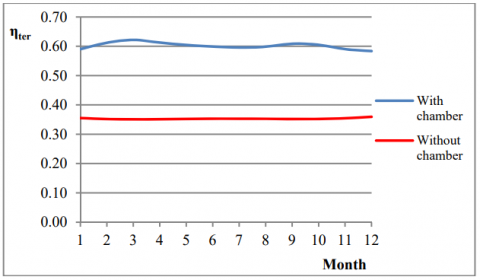Figure 14. Thermal efficiency of the system (Ass_SiC)

## 15.png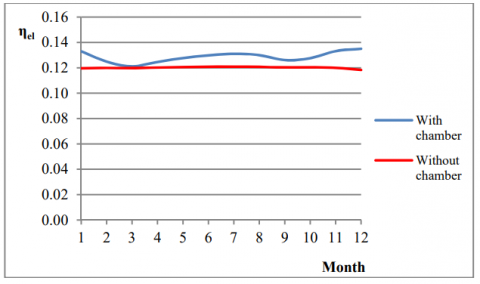Figure 15. Electrical efficiency of the system (Ass_SiC)

## 16.pngFigure 16. Total efficiency of the system (Ass_SiC)

## 17.png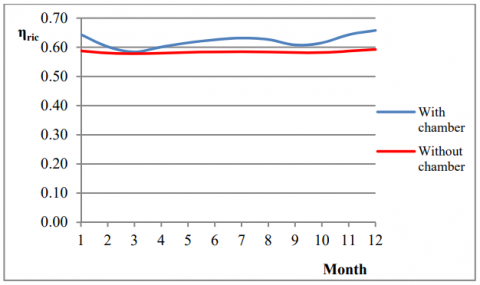Figure 17. Efficiency of the absorber (Ass_SiC)

Table 8. Mean values of Tass, Pa_ric, Qu, Pel, Pter (Ass_SiC)

 Model Tass [°C] Pa_ric   [W] Qu [W] Pel   [W] Pter   [W] With hot chamber 561.65 6881.62 4531.46 1076.62 5044.77 Without hot chamber 539.05 6609.41 4286.18 1011.87 2974.31

Table 9. Mean values of ηter, ηel, ηg,IP, ηg,IIP, ηric (Ass_SiC)

 Model ηter ηel ηg,IP ηg,IP ηric With hot chamber 0.602 0.129 0.731 0.520 0.621 Without hot chamber 0.353 0.120 0.474 0.350 0.584

Table 10. Mean values of ηter,m, ηel,m, ηg,m,IP, ηg,m,IIP (Ass_SiC)

 ηter,m ηel,m ηg,m,IP ηg,m,IIP Mean values With hot chamber 0.696 0.238 0.934 0.690 Without hot chamber 0.694 0.236 0.930 0.687

While at the level of the engine, the presence of the hot chamber seems not to bring significant benefits in that it causes differences on efficiencies less than 1% (Tables 7 and 10), this is no longer true at the level of the overall system. For the latter, in fact, the use of a hot chamber with absorber

in stainless steel involves an increase of 14% on electrical efficiency, of 75% on thermal efficiency and of 54% on the total efficiency of second law of thermodynamics, compared to the same plant with simple absorber.

Table 11. Percentage differences between the two plant solutions.

 Percentage differences Ass_Inox Ass_SiC Tass 8% 4% Pa_ric 18% 4% Qu 11% 6% Pel 13% 6% Pter 75% 70% ηter 75% 70% ηel 14% 7% ηg,IP 60% 54% ηg,IIP 54% 49% ηric 12% 6%

With the use, instead, of an absorber made of silicon carbide, the increments are, respectively, 7%, 70% and 49%.

Minor offsets are justified by the fact that the SiC, allowing a greater absorption of solar energy than

sandblasted stainless steel, involves absorber temperatures almost similar in the two solutions.

Obviously, the efficiency of the parabola is not influenced by the plant solution adopted, and it is 87%.

5. Conclusions

This paper analyses the performances of a Dish-Stirling concentrating solar system using the Stirling engine for the production of electrical and thermal energy. In particular, with focus on the receiver, its performance was evaluated considering two different system solutions: the first using the absorber with hot chamber and the second an absorber without hot chamber.

The results derived from simulations revealed that the use of the absorber provided with hot chamber entails a considerable increase in performance of the system, especially from a thermal point of view, owing to the further removal of the thermal power resulting from the cooling of the walls of the hot chamber. This system solution enables the temperature of the absorber to be kept always close to the maximum allowed value so that the engine can provide the nominal values of thermal and electric power.

With temperature control the possible irreversible deformations of the engine and the continuous misalignments of the parabola are averted.

In particular, for a system with a parabola of 10 m2 area, absorber in stainless steel and a Stirling engine of 1 kWe, located in Cosenza (Italy), the presence of the hot chamber entailed for the system an increase of 14% in the electrical efficiency, of 75% in thermal efficiency and of 54% in the total efficiency of the second law of thermodynamics, compared to the same plant with simple absorber.

The use of an absorber made of silicon carbide and hot chamber involves, however, an increase of 7% in electrical performance, of 70% in thermal efficiency and of 49% in the total efficiency of the second law of thermodynamics.

References

1. S. Cucumo, A. Muzaka, L. Laino, E. Pastorelli, “Analisi delle prestazioni di un sistema solare termico a concentrazione (csp) con stima della producibilità,” Atti del 7° Congresso Nazionale AIGE, Rende (CS), 10-11 Giugno 2013. Edizioni TEOMEDIA.

2. M.F. Modest, Radiative Heat Transfer, McGraw-Hill, New York, 1993.

3. ASHRAE Handbook-Fundamentals, New York, 1989.

4. M.A. Cucumo, D. Kaliakatsos, V. Marinelli, Energetica, Pitagora Editrice, Bologna, 2006.